# Simulation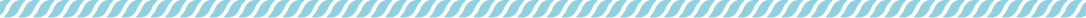# Fundamental differential equation

Now, we will discuss how well SLUGGER can adsorb the influenza virus. This is the simulation of SLUGGER as a medicine. We define X as the amount of virus adsorbed in some way. When considering the reaction kinetics, the amount of adsorption is given by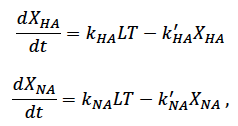where k and k’ is the reaction rate constant, L is an amount of SLUGGER and T is an amount of the influenza viruses. The subscripts of X, k and k’ indicate which spike they are connected. k is the reaction rate constant of adsorption and k’ is the reaction rate constant of desorption. The amounts of L and T are defined in terms of concentration(mol/L). Because X is a part of T0 which means the initial concentration of viruses, X/T0<1 will be always true. Then, if L>>T, which means that the concentration of SLUGGER is far higher than that of virus, is true, L is nearly equal to L0 which means the initial concentration of SLUGGER.
The relationship of T, T0 and X is T0 = T + X, so we can substitute T0-X in T and X equals to the sum of  XHA and XNA. Assuming a state of equilibrium, both  dXHA/dt and dXNA/dt equal to 0. From these conditions, the two equations can be rewritten as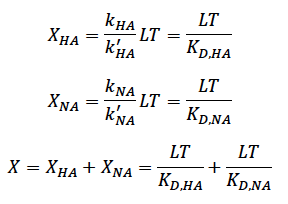Then, we define f as the ratio of the amount of virus bound to the SLUGGER in some way at equilibrium to the initial concentration of the virus. This is the percentage of virus that is partially covered by SLUGGER and is expected to inhibit binding to cells. To calculate f, the equation can be rearranged to yield,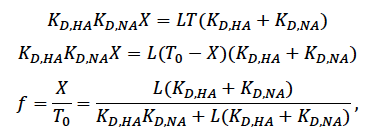where KD is dissociation constant.

# Correction for the surface density of HA and NA

Since the ratio of HA to NA is 4:1, the probability of a SLUGGER colliding with SLUGGER is determined by that ratio. So, the differential equation is rewriting as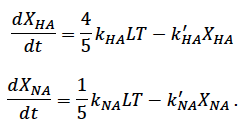In this case, f is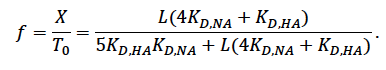The results of calculation are following,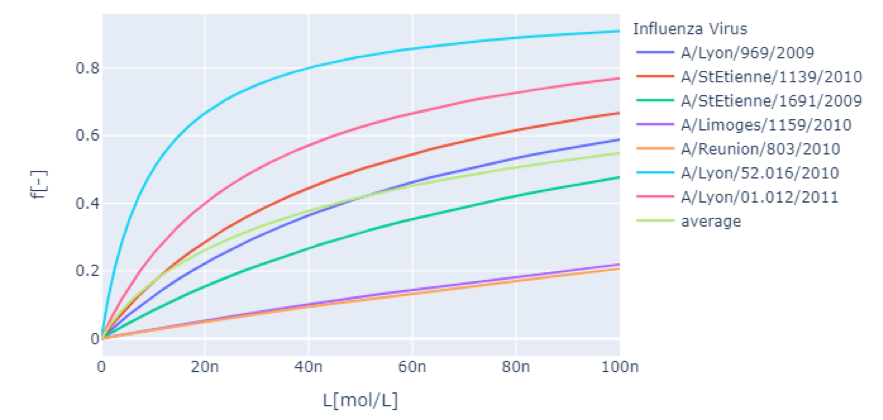Figure 1 : The result of the former
f is the ratio of SLUGGER-bound virus to the initial concentration of virus. L is the concentration of SLUGGER. n means nano, for example 100n = 100 × 10^(-9).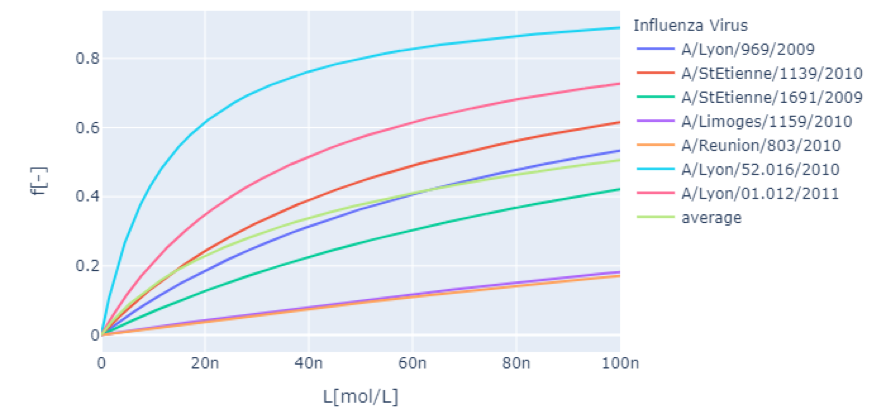Figure 2 : The result of the latter
f is the ratio of SLUGGER-bound virus to the initial concentration of virus. L is the concentration of SLUGGER. n means nano, for example 100n = 100 × 10^(-9).

We used KD values of sialylglycopolymer. However, because we tackled to make the structure like sialic acid on a plate, the whole structure is different.This difference changes the KD values. Therefore, it needs to be corrected in some way. We have attempted to supplement the values from the measured adsorption and changes in the dissociation constant.We have referred to three experimental facts; first, ’The amount of adsorption increased by 0.6 times by changing from O to S’; second, ‘When the distance between sialic acids was 59Å, the bond dissociation constant was 0.121 times higher than that with a single sialic acid’; third, A 10-fold increase in the binding constant in the AMP bilayer. These experimental facts were simply multiplied by the bonddissociation constant, and this was taken as the new dissociation constant; the third fact does not involve any sialic acid, so it is of course a leap of logic to use this data, but since no similar data existed or could be found, which we will use. For the second experimental fact, since the value indicated with two sialic acids cooperate, we divided it by two in the correction factor and converted it to per strand.So, new KD, KD’ is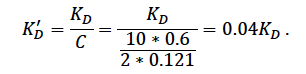The results of calculation are shown the following two figures.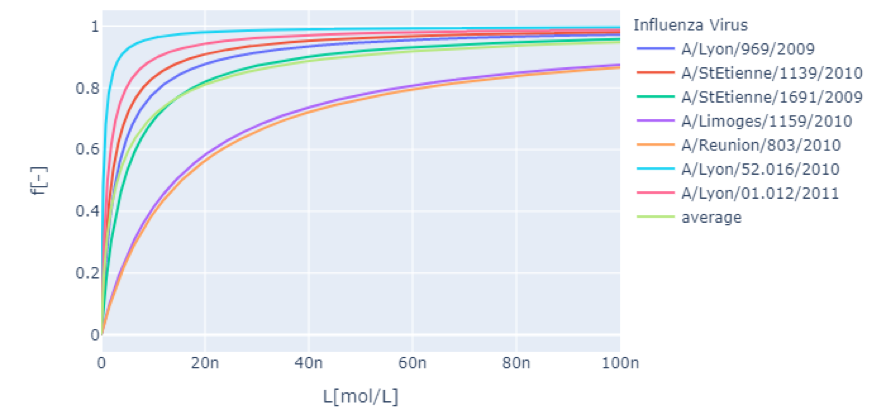Figure 3 : The result of the former with correction factor
f is the ratio of SLUGGER-bound virus to the initial concentration of virus. L is the concentration of SLUGGER. n means nano, for example 100n = 100 × 10^(-9).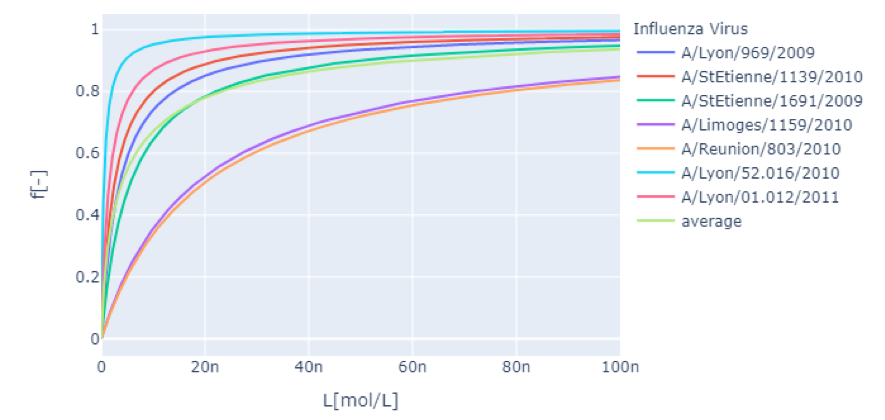Figure 4 : The result of the latter with correction factor
f is the ratio of SLUGGER-bound virus to the initial concentration of virus. L is the concentration of SLUGGER. n means nano, for example 100n = 100 × 10^(-9).

# Findings from the calculations

These results of calculation indicate that the amount of virus that can be adsorbed depends not only on the concentration of SLUGGER but also on the type of virus strain. When we add SLUGGER to make the 100 nmol/L SLUGGER solution without correction factor, over 90% of A/Lyon/52.016/2010 is captured, however, the ratio of A/Reunion/803/2010 and A/Limoges/1159/2010 is only 20%. These results indicate that there are certain virus strains that can be effective and that there are some virus strains that have little potential to be effective.

# The problem of the calculation

This calculation is the simplest model for analyzing adsorption, so we ignored the complexity of the mechanism of adsorption and the behavior of molecules and viruses. We corrected the dissociation constant using a correction factor, which is a simple product of the experimental facts. Although the experimental facts themselves are unquestionable, a simple multiplication of them is not supported by the theory. The analysis by chemical kinetics could be inappropriate because it is a macroscopic analysis of matter and rate constants may be completely different when microstructure is changed. Molecular dynamics simulation is one of the methods to solve this problem, but we could not tackle it due to our lack of knowledge, technology, and computational resources.
In the case of actually using in bodies, the f value is expected to be lower than that shown here because SLUGGER will compete with sialic acid on the cell surface. If the amount of sialic acid on the cells is as same as SLUGGER, the effect is not expected high because sialic acid can be cleaved by NA, so desorption more frequently occurs. However, because the actual amount of sialic acid that can be related is unknown and thought that it is not low, its effect on f value is expected high.

# Ground Simulation

Simulations were performed using the Cando software on the caDNAno designs discussed in the section of DNA origami design. The examined models are 1 With Stopper, 2 Without Stopper.

1.     With Stoppers: Stopper is single-stranded
2.     Without Stoppers: Stopper forms double strands

The color bar under the figure reflects the calculated values for the stability of the DNA origami, and the smaller the value, the more stable the structure is.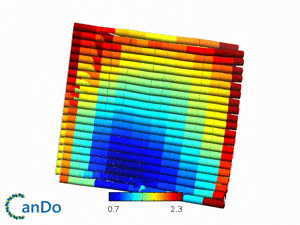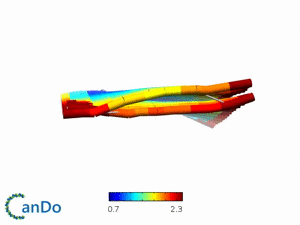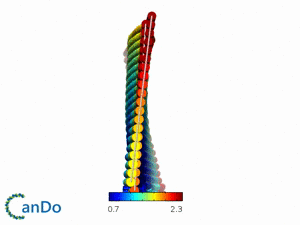Figure 5 : The result of  "1. With Stoppers"
Hinge is folded by the stoppers and the surface area is smaller than one without stoppers.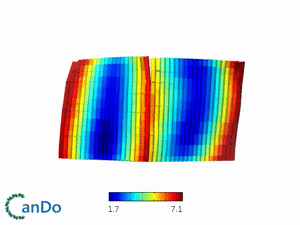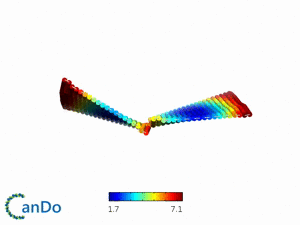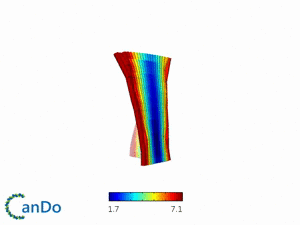Figure 5 : The result of  "2. Without Stoppers"
Hinge is unfolded and creates flexible rotational motion.The surface area is larger than one with stoppers.

# Reference

 Jean-Sébastien Casalegno, Olivier Ferraris, Vanessa Escuret, Maude Bouscambert, Corinne Bergeron, Laetitia Linès, Thierry Excoffier, Martine Valette, Emilie Frobert, Sylvie Pillet, Bruno Pozzetto, Bruno Lina, Michèle Ottmann, PLoS One. 2014; 9(8): e104009.

 Yasuo Suzuki, Katsuhiko Sato, Makoto Kiso, Akira Hasegawa, Glycoconjugate J (1990) 7:349-356.

 Victor Bandlow, Susanne Liese, Daniel Lauster, Kai Ludwig, Roland R. Netz, Andreas Herrmann, Oliver Seitz, J. Am. Chem. Soc. 2017, 139, 45, 16389–16397.

 Mitsuhiko Onda, Kanami Yoshihara, Hiroshi Koyano, Katsuhiko Ariga, Toyoki Kunitake, J. Am. Chem. Soc. 1996, 118, 8524-8530.

©︎ BIOMOD JAPAN OPEN 2020 Team E